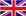English
Blog

### The general concept of current transformers

August 06 , 2020

A general current transformer can transform a primary current with a large value into a secondary current with a smaller value according to a certain transformation ratio, so as to play the main purpose of maintenance and accurate measurement. The main function of the zero-sequence current transformer is to protect the power circuit when an electric shock accident or common leakage occurs in the power circuit, and to disconnect the switching power supply. Although the names of these two transformers are very similar, they have great effects. Not the same, what are their differences and main functions actually? The core-through current transformer is no problem in terms of working principle as a zero-sequence transformer, but the sensitivity of the transformer will be very different.

1. The main function of general current transformer

Generally, a current transformer can transform a primary current with a large value into a secondary current with a smaller nominal value according to a certain transformation ratio, so as to play the main purpose of maintenance and accurate measurement. For example, a current transformer with a transformation ratio of 400/5 can change a current of 400A into a current of 5A.

2. The working principle of zero sequence transformer

The working principle of zero sequence current maintenance is based on Kirchhoff's current law: the algebraic sum of the complex current injected into any connection point in the power circuit is equal to zero. Under normal conditions of the line and electrical equipment, the vector sum of the currents of each phase is equal to zero, so the secondary winding of the zero-sequence current transformer has no signal output, and the execution components will not carry out actions. When common faults of grounding devices occur, the vector sum of the currents of each phase is not zero. Common fault currents will cause magnetic flux in the toroidal core of the zero-sequence current transformer, and the induced voltage on the secondary side of the zero-sequence current transformer will cause the execution The components carry out actions to drive the tripping equipment and switch the power supply network to achieve the purpose of ground fault protection.

3. What is the function of zero sequence transformer

When an electric shock accident or a common fault occurs in the power circuit, it can play a protective action and disconnect the switching power supply.

4. What are the use conditions of zero sequence transformer

Everyone can install a current transformer on the three-phase line, or let the three-phase transmission line cross the zero sequence current transformer together. Of course, you can also install a zero sequence current transformer on the neutral point N. The zero-sequence current transformer can be used to check the three-phase current vector sum.

The actual application of zero-sequence current protection can be to install a current transformer (CT) on each of the three-phase lines, or let the three-phase transmission line cross the zero-sequence CT together, and also install a zero-sequence CT on the neutral point N. In this case We can use this zero-sequence CT to check the three-phase current vector sum, that is, the zero-sequence current Io, IA+IB+IC=IO, when the three-phase load connected to the line is completely equivalent (common in the absence of grounding devices) Failure, and without considering the leakage current of the line and electrical equipment), IO=0; when the three-phase load connected to the line is unbalanced, then IO=IN, the zero sequence current at this time is the unbalanced current IN; When a common fault of the grounding device occurs in one phase, a common fault current Id for single-phase grounding will definitely be generated. At this time, the zero sequence current IO=IN+Id tested by your friends is actually three-phase unbalanced current and single-phase grounding current The vector sum.

#### Tags

welcome to ZTC
Talk To ZTC Today To Discuss Your Need For Current Transformers,Get Standard Item, OEM/ODM Service Quote Are Available.